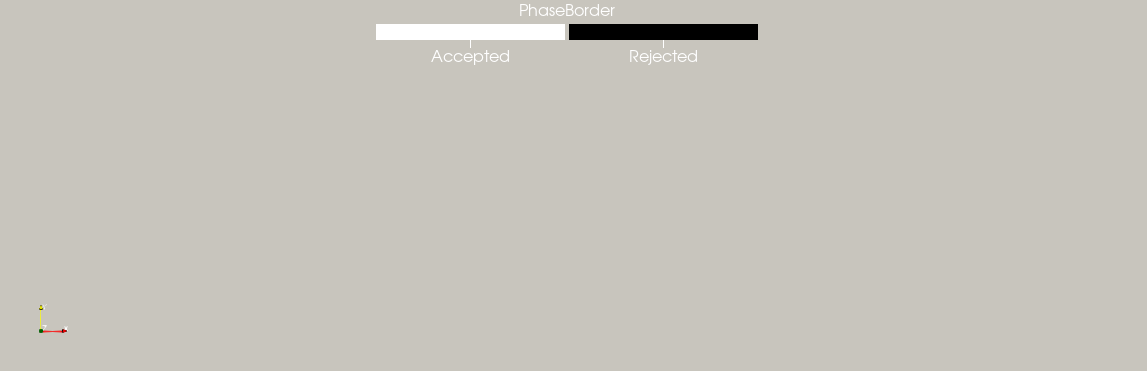# Pixels at the edge of the screen

The focal length of the camera used in Polymap is really short so when the camera sees the fringes by reflection on the surface, they appear as blurred.

If the fringes are large enought in comparison to the size of the blur, it does not have a huge impact, we can recognize the shape of a sinus. However for edges pixels, the intensity and the contrast drops quickly.

To find those corrupted pixels, we need to find the minimum and maximum values phases can have and find the pixels whose phase is not in these interval

``````double margin = 0.5; (1)

// Horizontal
int nbFringeH = (ncols_ )/fringeLenH ;
auto phaseMinH = - (nbFringeH-margin) * M_PI;
auto phaseMaxH = (nbFringeH-margin) * M_PI;
// Vertical
int nbFringeV = (nrows_ )/fringeLenV ;
auto phaseMinV = - (nbFringeV-margin) * M_PI;
auto phaseMaxV = (nbFringeV-margin) * M_PI;

DphaseBorder_ = ( (px.array() > phaseMinH) && (px.array() < phaseMaxH)
&& (py.array() > phaseMinV) && (py.array() < phaseMaxV) ).select(matrix_1, matrix_0); (2)``````
 1 Small margin to make sure pixels are not exactly at the border of the screen 2 Selection
 With the current default settings of Polymap, this indicator either overlaps the previous ones and will have no effects or doesn’t detect any pixel at all. However this indicator might be helpful if the focal length or the resolution of the camera changes. In later projects such as Holomap, this indicator might become relevant.Figure 1. Empty indicator